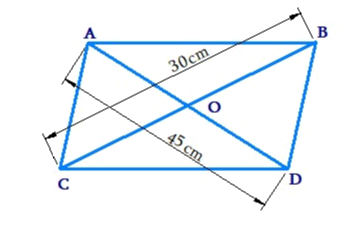# Ex.11.2 Q7 Mensuration Solution - NCERT Maths Class 8

Go back to  'Ex.11.2'

## Question

The floor of a building consists of $$3000$$ tiles which are rhombus shaped and each of its diagonals is $$45 \,\rm{cm}$$ and $$30\,\rm{ cm}$$ in length. Find the total cost of polishing the floor, if the cost per $$\rm{m}^2$$ is $$4.$$

Video Solution
Mensuration
Ex 11.2 | Question 7

## Text Solution

What is Known?

The tiles used are rhombus shaped. Dimensions of single tile used and cost per $$\,{m^2}$$ of polishing the floor.

What is unknown?

Total cost of polishing the floor.

Reasoning:

By using the method of splitting into triangles (triangulation method) we can find area of the rhombus visually diagonals $$AD$$ and $$BC$$ are perpendicular bisectors of each other hence area of rhombus $$ABCD$$ will be the sum of area of triangle $$ACB$$ and area of the triangle $$DBC.$$

Steps:Area of rhombus

\begin{align}& = \! {\text{Area of }}\Delta ABC \! + \! {\text{Area of }}\Delta DCB\\& = \! \frac{1}{2} \! \times \! (BC \! \times \! AO) \! + \! \frac{1}{2} \! \times \! (BC \! \times \! OD)\\& = \! \frac{1}{2} \! \times \! BC \! \times \! (AO \! + \! OD)\\&= \! \frac{1}{2} \! \times \! BC \! \times \! AD\\&= \! \frac{1}{2} \! \times \! 45\,{\rm{cm}} \! \times \! 30\,{\rm{cm}}\\& = \! 675\,{\rm{c}}{{\rm{m}}^2}\\\end{align}

Area of rhombus $$ABCD$$

\begin{align} &= \! \text{Area of }\Delta ABC \! + \! {\text{Area of }}\Delta DCB\\ &= \! \frac{1}{2} \! \times \! (BC \! \times \! AO) \! + \! \frac{1}{2} \! \times \! (BC \! \times \! OD)\\&= \! \frac{1}{2} \! \times \! BC \! \times \! (AO \! + \! OD)\\& = \! \frac{1}{2} \! \times \! BC \! \times \! AD\\&= \! \frac{1}{2} \! \times \! 45\,{\rm{cm}} \! \times \! 30\,{\rm{cm}}\\& = \! 675\,{\rm{c}}{{\rm{m}}^2}\end{align}

Area of each tile $$= 675\,\rm{m^2}$$

Area covered by $$3000$$ tiles

\begin{align}& = (675 \times 3000){\rm{c}}{{\rm{m}}^2}\\& = 2025000\,{\rm{c}}{{\rm{m}}^2}\\ &= 202.5\,{{\rm{m}}^2}\end{align}

The cost of polishing is $$\rm{Rs.}\, 4$$ per $${m^2}$$.

$$\therefore$$ Cost of polishing for $$202.5\,\rm{m^2}$$area $$= \rm{Rs.}(4 \times 202.5) = \rm{Rs.}810.0$$

Thus, the cost of polishing the floor is $$\rm{Rs }\,810.$$

Learn from the best math teachers and top your exams

• Live one on one classroom and doubt clearing
• Practice worksheets in and after class for conceptual clarity
• Personalized curriculum to keep up with school